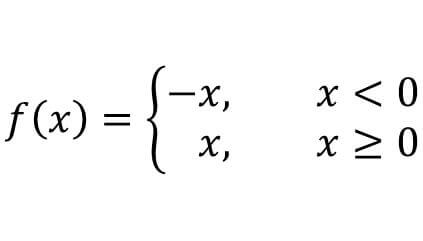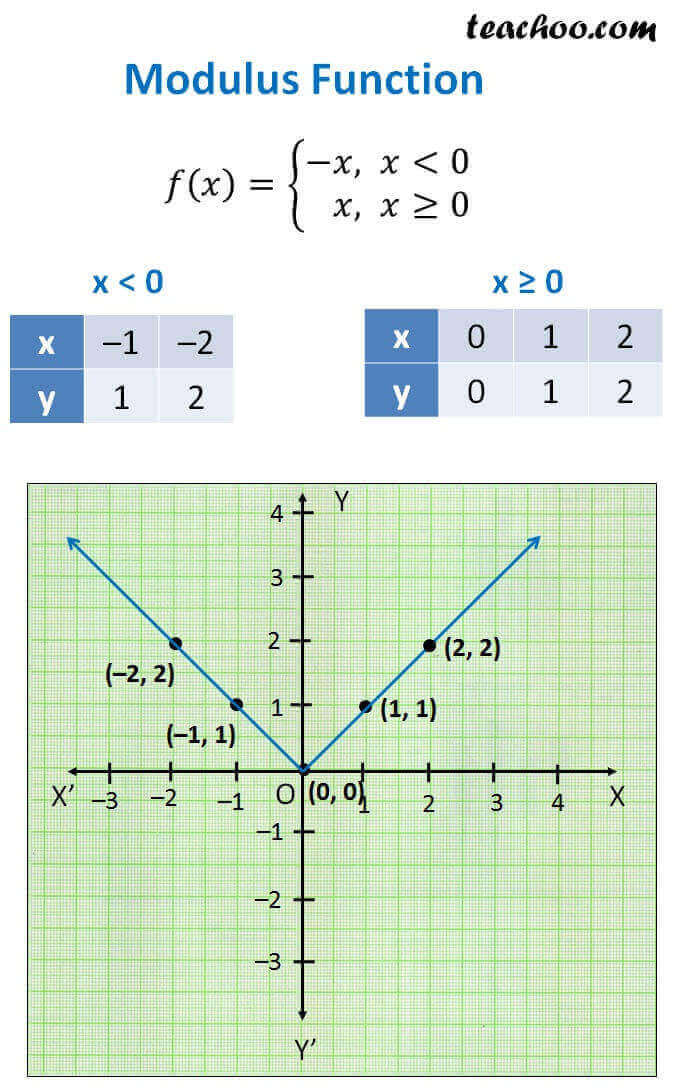Different Functions and their graphs

Chapter 2 Class 11 Relations and Functions
Concept wise

f: R R

f(x) = |x| for each x ∈ R

Here |x| is known as modulus of x

|x| is always positive.

So, |–1| = 1

|–2| = 2

|1| = 1

|2| = 1

|0| = 0

Let us rewrite the functionLet’s check for different values of x

#### For x = –1

x < 0

So, f(x) = – x

f(x) = – (–1) = 1

#### For x = –2

x < 0

So, f(x) = – x

f(x) = – (–2) = 2

x > 0

So, f(x) = x

f(x) = 1

x > 0

So, f(x) = x

f(x) = 2

#### For x = 0

x ≥ 0

So, f(x) = x

f(x) = 0

Now,

Plotting graphHere,

Domain = All values of x = R

Range = All values of y

Since y will always be positive or 0

Range = All positive Real numbers and 0

Get live Maths 1-on-1 Classs - Class 6 to 12